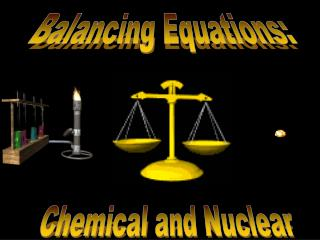DownloadDownload PresentationBalancing Equations:

# Balancing Equations:

Télécharger la présentation## Balancing Equations:

- - - - - - - - - - - - - - - - - - - - - - - - - - - E N D - - - - - - - - - - - - - - - - - - - - - - - - - - -
##### Presentation Transcript

1. Balancing Equations: Chemical and Nuclear

2. Ca O O H H How molecules are symbolized Cl2 2Cl 2Cl2 • Molecules may also have brackets to indicate numbers of atoms. E.g. Ca(OH)2 • Notice that the OH is a group • The 2 refers to both H and O • How many of each atom are in the following? a) NaOH b) Ca(OH)2 c) 3Ca(OH)2 Na = 1, O = 1, H = 1 Ca = 1, O = 2, H = 2 Ca = 3, O = 6, H = 6

3. Mg Mg O O O  + Balancing equations: MgO • The law of conservation of mass states that matter can neither be created or destroyed • Thus, atoms are neither created or destroyed, only rearranged in a chemical reaction • Thus, the number of a particular atom is the same on both sides of a chemical equation • Example: Magnesium + Oxygen (from lab) • Mg + O2 MgO • However, this is not balanced • Left: Mg = 1, O = 2 • Right: Mg = 1, O = 1

4. From Mg + O2 MgO 2Mg + O2 2MgO is correct Mg + ½O2 MgO is incorrect Mg2 + O2 2MgO is incorrect 4Mg + 2 O2 4MgO is incorrect Balance equations by “inspection” Hints: start with elements that occur in one compound on each side. Treat polyatomic ions that repeat as if they were a single entity. 5 a) P4 + O2 P4O10 b) Li + H2O  H2+ LiOH c) Bi(NO3)3 + K2S Bi2S3 + KNO3 d) C2H6 + O2 CO2 + H2O 2 2 2 2 3 6 3.5 2 3 C2H6 + O2 CO2 + H2O 2 7 4 6

5. Balance these skeleton equations: a) Mg + 2HCl  MgCl2 + H2 b) 3Ca + N2 Ca3N2 c) NH4NO3 N2O + 2H2O d) 2BiCl3 + 3H2S  Bi2S3 + 6HCl e) 2C4H10 + 13O28CO2 + 10H2O f) 6O2 + C6H12O66CO2 + 6H2O g) 3NO2 + H2O 2HNO3 + NO h) Cr2(SO4)3+ 6NaOH  2Cr(OH)3+ 3Na2SO4 i) Al4C3 + 12H2O 3CH4 + 4Al(OH)3

6. Returning to reaction types • We have looked at several types of reactions without worrying about balancing • However, all equations should be balanced • Predict the products and balance these: (recall, metals above replace metals below, reactionswithwateryieldmetalhydroxides) Cu + Fe2(SO4)3 2 3 3 Fe + CuSO4  Ni + NaCl  NR (no reaction) 2 3 3 2 Al + CuCl2  Cu + AlCl3 Zn + Li2CO3 Li + ZnCO3  2 2 2 2 ½ LiOH + H2 Li + H2O  Al + O2  4 3 2 Al2O3

7. Phosphorescent zinc sulfide detection screen Lead block +   Radioactive substance  – Strong magnetic or electrostatic field Discovery of Radioactivity • Radioactivity is the release of energy or particles when an atom disintegrates (demo) • Radioactivity was discovered when minerals were exposed to film through an opaque cover • The 3 types of radioactivity can be shown by passing emissions through an electrical field:

8. Types of Radioactivity Types of radiation: 1)a, 2) b, 3) • Alpha () particles are symbolized as 42He • Beta()particles(essentiallyelectrons)are0–1e • Gamma (g) rays are symbolized as 00 • You can determine the composition of each: a: mass of 4 u, charge of +2 (2 p+, 2 n0, 0 e–) • Other symbols: proton = 11p, neutron = 10n • There are different terms to describe the different types of nuclear reactions • “alpha decay” means an a particle is given off. • Other: beta decay, fusion (meaning to bring together), fission (meaning to break apart)

9. 235 1 1 139 +  + U n 3 + n Ba 0 92 0 56 Nuclear equations 14 0 14  + C e N 6 7 -1 Q. Write the beta decay for C-14 Q. Write the alpha decay for 209Po 209 4 205  + Po He Pb 84 2 82 Q. Complete this fission reaction 94 Kr 36 • In all cases, charge and mass must be balanced • Practice: pg. 222-3, Q6, Q3

10. 99 4 32 99 187 Tc He P Sr Re 15 43 2 38 75 226 4 222  + Ra He Rn 88 2 86 84 0 84  + Br e Kr 35 -1 36 242 4 238  + Pu He U 94 2 92 14 18 1 4 11 +  + p O N He C 1 7 8 2 6 0 1 19 +  e + n F 0 -1 9 • a) • b) • c) • d) • e) 6. a) b) c) d) e)

11. Here are some more to balance: a) 2KNO32KNO2 + O2 b) 2Pb(NO3)22PbO + 4NO2 + O2 c) P4 + 6I24PI3 d) 3MgO + 2H3PO4 Mg3(PO4)2 + 3H2O e) Br2 + 2KI  I2 + 2KBr f) Ca(OH)2 + 2HNO3 Ca(NO3)2 + 2H2O g) Bi2O3 + 3H22Bi + 3H2O h) 3Fe + 2O2 Fe3O4 i) 2CaO + 5C 2CaC2 + CO2

12. Question 3 pg. 252 a) 2Li + 2H2O ® H2 + 2LiOH b) P4 + 5O2® P4O10 c) 2C2H6 + 7O2® 4CO2 + 6H2O d) CS2 + 3O2® CO2 + 2SO2 e) 2AsCl3 + 3H2S ® As2S3 + 6HCl f) 3AgNO3 + FeCl3® 3AgCl + Fe(NO3)3 g) 2KClO3® 2KCl + 3O2 h) 2SO2 + O2® 2SO3 For more lessons, visit www.chalkbored.com